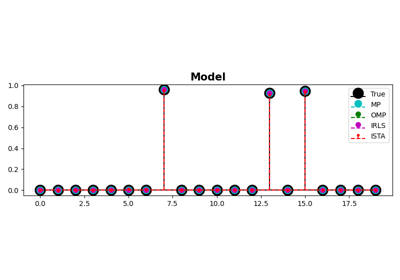# pylops.optimization.sparsity.omp#

pylops.optimization.sparsity.omp(Op, y, niter_outer=10, niter_inner=40, sigma=0.0001, normalizecols=False, show=False, itershow=(10, 10, 10), callback=None)[source]#

Orthogonal Matching Pursuit (OMP).

Solve an optimization problem with $$L^0$$ regularization function given the operator Op and data y. The operator can be real or complex, and should ideally be either square $$N=M$$ or underdetermined $$N<M$$.

Parameters
Oppylops.LinearOperator

Operator to invert

ynumpy.ndarray

Data

niter_outerint, optional

Number of iterations of outer loop

niter_innerint, optional

Number of iterations of inner loop. By choosing niter_inner=0, the Matching Pursuit (MP) algorithm is implemented.

sigmalist

Maximum $$L_2$$ norm of residual. When smaller stop iterations.

normalizecolslist, optional

Normalize columns (True) or not (False). Note that this can be expensive as it requires applying the forward operator $$n_{cols}$$ times to unit vectors (i.e., containing 1 at position j and zero otherwise); use only when the columns of the operator are expected to have highly varying norms.

showbool, optional

Display iterations log

itershowtuple, optional

Display set log for the first N1 steps, last N2 steps, and every N3 steps in between where N1, N2, N3 are the three element of the list.

callbackcallable, optional

Function with signature (callback(x)) to call after each iteration where x is the current model vector

Returns
xinvnumpy.ndarray

Inverted model

niter_outerint

Number of effective outer iterations

costnumpy.ndarray

History of cost function

ISTA

Iterative Shrinkage-Thresholding Algorithm (ISTA).

FISTA

Fast Iterative Shrinkage-Thresholding Algorithm (FISTA).

SPGL1

Spectral Projected-Gradient for L1 norm (SPGL1).

SplitBregman

Split Bregman for mixed L2-L1 norms.

Notes

## Examples using pylops.optimization.sparsity.omp#MP, OMP, ISTA and FISTA

MP, OMP, ISTA and FISTA